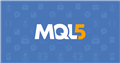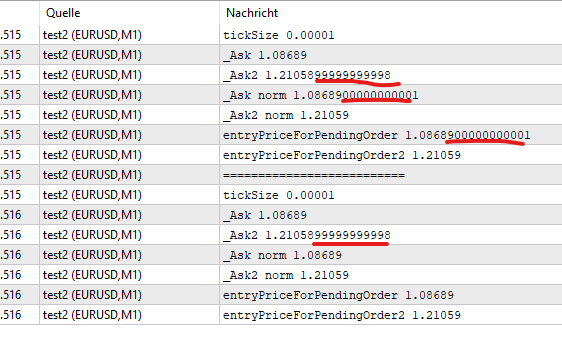# How to handle price calculations for different asset classes?

Hi,

I want to run an EA in strategy tester with different symbols/asset-classes at once, therefore I want to have one set of optimization settings for all assets. Is that possible somehow?

For example (all just sample numbers!):

ATR has a value of 16
SL needs to be 20 point below entry, so entryPrice - 20
...

ATR has a value of 0.00024
SL needs to be 20 Pips below entry , so entryPrice - 0.0020
...

ATR has a value of 0.15
SL needs to be 2 Pips below entry , so entryPrice - 2
...

If we talk about Cryptopairs... you know what I write here....

So, how to handle this differences? Do I have to have different settings for every asset class or do I have to distinguish in the EA code somehow? I know there are things like TICK_SIZE, TICK_VALUE, Point()... but I don't get it in the brain how to handle this. Do I have to reduce every calculation on a base of money, I#m willing to loose/win?

How do YOU handle this, to get an multi-asset-class EA? I'm even not sure if my question makes sense at all, may be I'm on the wrong way or don't understand sth. - then please correct me.

Thanks a lot,
Alex

To improve your EA to be able to work with all asset classes, you should do away with idea of pips, and work with "tick size" and "tick value" only.

If you wish to use "points", you can but be careful to always convert it every time and to normalise or adjust it to the tick size as well.

This is because there are asset classes where the "point size" and "tick size" are different. Best to stick to just using the "tick size" and "tick value".

Symbol Point Value

Fernando Carreiro, 2022.06.02 01:14

Here are two examples from AMP Global (Europe):

• Micro E-mini S&P 500 (Futures): point size = 0.01, tick size = 0.25, tick value = \$1.25
• EURO STOXX Banks (Stock Index): point size = 0.01, tick size = 0.05, tick value = €2.50

Symbol Point Value

Fernando Carreiro, 2022.05.18 21:05

```double
dbTickSize   = SymbolInfoDouble( _symbol, SYMBOL_TRADE_TICK_SIZE  ), // Tick size
dbTickValue  = SymbolInfoDouble( _symbol, SYMBOL_TRADE_TICK_VALUE ), // Tick value
dbPointSize  = SymbolInfoDouble( _symbol, SYMBOL_POINT ),            // Point size
dbPointValue = dbTickValue * dbPointSize / dbTickSize;               // Point value
```
Remember, it's best to use tick size and tick value in your calculations, instead of point size and its value.

Tick size vs Point(), can be a little tricky in Multicurrency EA

Fernando Carreiro, 2022.03.09 12:11

Tick Size and Point Size can be very different especially on stocks and other symbols besides forex.

Always use Tick Size to adjust and align your prices, not the point size. In essence, make sure that your price quotes, are properly aligned to the Tick size (see following examples).

```...
double tickSize = SymbolInfoDouble( _Symbol, SYMBOL_TRADE_TICK_SIZE );
...
double normalised_price = round( price / tick_size ) * tick_size;
...
// Or use a function
double Round2Ticksize( double price )
{
double tick_size = SymbolInfoDouble( _Symbol, SYMBOL_TRADE_TICK_SIZE );
return( round( price / tick_size ) * tick_size );
};```

NormalizeDouble not "working"

Fernando Carreiro, 2017.09.23 00:41

Just as a side note.

Initially, when I started with MQL, I would manipulate prices as "doubles". Nowadays, especially in the more complex EA's, I manipulate prices as "ints". At the very first opportunity, I convert a price into ticks:

`int priceTicks = (int) round( price / tickSize );`

From then on, all my calculations and manipulations are done with "ints". Not only is it more memory compact and much faster, but comparisons are much easier to handle.  Doing a "priceA == priceB" for "doubles" is quite problematic, but not for "ints" because it gives exact matches. Not to mention, that in this way prices, stop sizes, etc. are ALWAYS aligned.

Then, just before I have to place or modify an order, I then convert it back:

`priceTicks * tickSize`
EDIT: I do the same for volume/lots by using the broker's Lot-Step.Documentation on MQL5: Checkup / Point
• www.mql5.com
Point - Checkup - MQL5 Reference - Reference on algorithmic/automated trading language for MetaTrader 5

If I understand you correctly, I would do this:

```input double inpAtrMultiplier = 1.5;

void whereYouCalculateStopLoss()
{
//double atr = ...
//double entryPrice = ...
double stopLoss = NormalizeDouble(entryPrice - slSize(atr), _Digits);
}

double slSize(double atr)
{
return(atr * inpAtrMultiplier);
}```

P.S. Instead of a NormalizeDouble(), you can normalize the price as Fernando said

Since @Fernando Carreiro suggestions sound interesting to me, I fiddled around a bit with that and came across the following: the printed doubles are somehow weird, why does it happen? NormalizeDouble(double, int) does the job.

```void OnInit() {

Print("tickSize " + tickSize);

int priceTicks = (int) round(_Ask / tickSize);
int priceTicks2 = (int) round(_Ask2 / tickSize);
// calculateSth();
Print("entryPriceForPendingOrder " + (priceTicks * tickSize));
Print("entryPriceForPendingOrder2 " + (priceTicks2 * tickSize));

Print("==========================");
Print("tickSize " + NormalizeDouble(tickSize, _Digits));

// calculateSth();
Print("entryPriceForPendingOrder " +  NormalizeDouble(priceTicks * tickSize, _Digits));
Print("entryPriceForPendingOrder2 " +  NormalizeDouble(priceTicks2 * tickSize, _Digits));

}```Alex #:

Since @Fernando Carreiro suggestions sound interesting to me, I fiddled around a bit with that and came across the following: the printed doubles are somehow weird, why does it happen? NormalizeDouble(double, int) does the job.

`DoubleToString()`

For converting to a string

Alex #: somehow weird, why does it happen? NormalizeDouble(double, int) does the job.

You used NormalizeDouble, It's use is usually wrong, as it is in your case.

1. Floating point has a infinite number of decimals, it's your not understanding floating point and that some numbers can't be represented exactly. (like 1/10.)
Double-precision floating-point format - Wikipedia, the free encyclopedia

2. Print out your values to the precision you want with DoubleToString - Conversion Functions - MQL4 Reference.

3. SL/TP (stops) need to be normalized to tick size (not Point) — code fails on non-currencies.
On 5Digit Broker Stops are only allowed to be placed on full pip values. How to find out in mql? - MQL4 programming forum (2011)

And abide by the limits Requirements and Limitations in Making Trades - Appendixes - MQL4 Tutorial and that requires understanding floating point equality Can price != price ? - MQL4 programming forum (2012)

4. Open price for pending orders need to be adjusted. On Currencies, Point == TickSize, so you will get the same answer, but it won't work on non-currencies. So do it right.
Trailing Bar Entry EA - MQL4 programming forum (2013)
Bid/Ask: (No Need) to use NormalizeDouble in OrderSend - MQL4 programming forum (2012)

5. Lot size must also be adjusted to a multiple of LotStep and check against min and max. If that is not a power of 1/10 then NormalizeDouble is wrong. Do it right.
(MT4 2013)) (MT5 2022))

6. MathRound() and NormalizeDouble() are rounding in a different way. Make it explicit.
MT4:NormalizeDouble - MQL5 programming forum (2017)
How to Normalize - Expert Advisors and Automated Trading - MQL5 programming forum (2017)

7. Prices you get from the terminal are already correct (normalized).

8. PIP, Point, or Tick are all different in general.
What is a TICK? - MQL4 programming forum (2014)